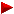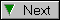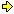HOME plateWON | World!OfNumbersFacts and figures about 2002the last palindromic year...that most of us will live to see!part 2year2002 part 1We live in 2002 and 878 years from now...
Are these two palindromes predicting a catastrophy !Will asteroid 1950DA hit the earth in 2880 ?
Note that 2880 = 2002 + 878, the sum of our two palindromes !

Read Amir Alexander's article from April 12, 2002A Close Call for Earth in 878 Years

The above link was sent to me by G. L. Honaker [email][site] (dd. 21/04/2002),
a loyal submitter to my website! Thanks.

(pdg) Not so long ago I saw a movie Armageddon  where a bunch
of oildiggers fixed another orbital bug. So I am confident for
the future. Palindromes (and humanity I hope) will survive 2880.Chocolate MathematicsEnoch Haga [email] forwarded me some fun maths (dd. 23/04/2002) -

" THIS IS THE ONLY YEAR (2002) IT WILL EVER WORK,
SO SPREAD IT AROUND WHILE IT LASTS. "

This is pretty neat how it works out.
This is cool chocolate math !!!!!!
DON'T CHEAT BY SCROLLING DOWN FIRST!
It takes less than a minute...
Work this out as you read.
Be sure you don't read the bottom until
you've worked it out! This is not
one of those waste of time things, it's fun.

1. First of all, pick the number of times a week that you would like
to have chocolate (try for more than once but less than 10).
2. Multiply this number by 2 (just to be bold).
4. Multiply it by 50. I'll wait while you get the calculator...
If you haven't, add 1751 ...
6. Now subtract the four digit year that you were born.
You should have a threedigit number...
The first digit of this was your original number
(i.e., how many times you want to have chocolate each week).
The next two numbers are...A 13 x 13 Magic Square with Magic Sum 2002...
(by Kishor N. Gordhandas from Bombay, India).
(email dd. 8/10/2002)The 169 cells of this super Magic Square are filled with all the integers
continuously from 70 up to 238.

 188 190 122 116 111 199 196 110 73 229 78 234 156 120 118 186 192 197 109 112 198 235 79 230 152 74 115 121 191 189 114 200 195 107 238 71 153 77 231 193 187 117 119 194 108 113 201 70 155 237 232 76 131 177 175 133 97 81 227 211 154 236 72 75 233 136 172 185 123 221 218 150 93 88 146 162 149 159 138 170 124 184 157 89 84 228 212 164 144 165 143 206 102 176 132 80 224 219 151 96 161 147 160 148 210 98 104 204 215 158 90 87 220 145 163 142 166 106 202 140 134 103 127 209 183 182 83 225 217 91 179 129 174 168 205 181 99 125 126 95 213 223 85 139 167 137 173 203 101 128 208 130 222 86 94 214 141 169 171 135 105 207 180 100 178 216 92 82 226

Some interesting peculiarities

This 13 x 13 = 169 Magic Square has a special number, namely  154 , which I call
the Magic Number. This number is common to many other smaller Magic Squares
inside this Super Magic Square.

There are the following smaller squares that you will find inside.
Four 4 x 4 = 16 squares with Magic Sum = (154 x 4) = 616 (top_left marked green)
Two 5 x 5 = 25 squares with Magic Sum = (154 x 5) = 770 (top_left = 73 & 97)
Two 7 x 7 = 49 squares with Magic Sum = (154 x 7) = 1078 (top_left = 114 & 175)
Two 9 x 9 = 81 squares with Magic Sum = (154 x 9) = 1386 (top_left = 111 & 131)
One 11 x 11 = 121 square with Magic Sum = (154 x 11) = 1694 (top_left = 115)
One main 13 x 13 = 169 square with Magic Sum = (154 x 13) = 2002
This is possible because 2002 = 2 x 7 x 11 x 13.

The top_left corners of the four 4 x 4 = 16 Magic Squares
with palindromic ! Magic Sum 616 are marked with a green background.

You will be able to find the other Magic sub_Squares quite easily.
ps. there exist one 3 x 3 = 9 square that is not perfect pandiagonal
since one of the diagonals sums to 465 instead of the Magic Sum 462.
154 marks the bottom_left corner of this square.

The main square has been drawn and inner squares have been
colourmarked wherever possible resulting in the file 'Magic 2002.jpg'.
All this was prepared for me by the use of the computer of my
artist friend. I hope everything is OK. This jpg file of 119 KB
is available by simple email request.

No doubt you will like and enjoy the square.A General Year 2002 Arithmetic Puzzle
(by Bruce A. Berentsen, Ph. D., Mathematician & Software Developer).

(email dd. 26/10/2002)Using integers 1 through 9 exactly once, and using either operators
+ or * between adjacent integers, develop an expression that yields
the value 2002.

a. Integers 1 through 9 are to be used exactly once, in any order.
b. Parentheses are permitted.
c. Integers used in the expression are not allowed to be a
concatenation of digits.
d. Only operators + and * are permitted between adjacent integers.

 Bruce's puzzle is a variation to a NPR Current Challenge given out dd. October 6, 2002 by Erich Friedman. Write out the digits from 1-9 in order. Then add some plus (+) signs and times (x) signs to the string to make it add up to 2,002. As usual in arithmetic, multiplication is done before addition, and you don't have to put a sign between every 2 digits. Answers 1 x 2 + 34 x 56 + 7 + 89 = 2002 and 1 x 23 + 45 x 6 x 7 + 89 = 2002

Solution
Bruce came out with the following two distinct solutions
without the use of a computer [ October 29, 2002 ].

1 * 2 * ( 5 + 6 ) * ( 3 * 4 + 7 + 8 * 9 ) = 2002 (courtesy of Bob Haymond)
and
(((( 9 + 7 ) * 5 + 3 ) * 4 * 6 ) + 2 + 8 ) * 1 = 2002 (by Bruce himself)

A computer program would have to be written to enumerate all possible solutions.
Who's candidate ?Terry Trotter (email dd. 04/01/2003)
writes that this digital topic is one of his favorites.
(it's like my All the King's Digits project "make a 100" in Math Forum.)
"Could 0 be included there, at least in the large-to-small order ?" he wonders...December 2002 is quite a month
(by Norma Smith, Universal Harmonics,
Master Analyst and Timekeeper).
(email dd. 5/12/2002)December = 55 (alpha)numerically, and is a Master Number or iterated
number such as 11, 22, 33, 99 and 111, 333, 888, 999. These calendar dates
are the numerical sum of a month + the date ( A=1, B=2, Z=26 ).
E.g. D-E-C-E-M-B-E-R = 4+5+3+5+13+2+5+18 = 55.

Months that have dates adding up to a Master Number of double digits
are 11 days apart... 11 + 11 = 22, + 11 = 33, etc. Notice this 11 theme
in the following data.

The 11 day Master Number cycles begin with December 1 to December 11th.
Thus, 55 + 11 = 66 as December 11. Eleven days later is 77, on Dec 22
( December as 55 + 22nd = 77 ).

Interesting that 23rd as 23 = End. It is the end of Master Number
cycles for this month and this year.

December 2002 is also the end of the unique 11 year Time Window from
1991 to 2002. These Mirror Years / Palindrome Years occur each century
110 years apart, thus the break in the 110 year cycle is unique.

I can verify the book end, flip-flop, opposites or polarity effect in
my life these past 11 years: major change has occured since I first
noticed the 1991 numerical oddity.

There is so much (esoteric!) interpretation that comes to the mind...More facts and figures of the palindrome 55 at WONplate 812002 at a palindromic position
in the decimal expansion of.

(PDG dd. 16/12/2002)The string 2002 was found at palindromic position 8622268 counting
from the first digit after the decimal point. The 3. is not counted.

Immediately we see that all the digits are even (2002 as 8622268).
The factorization of 8622268 = 2 * 2 * 373 * 5779 contains
another palindrome ! Note that 5779 - 2 - 2 = 5775 yet another palindrome !

Try to find the first palindromic positions in Pi for the following numbers :
or add entries yourself for whatever interesting reason...
313858 (equal length!), 632236
37324242 (smoothly undulating!)
1991?
20028622268 (all digits even)
2003?
2112?
8622268?

Find the first PRIME (+palindromic?) positions in Pi for the following numbers :
373?
1991?
2002?
2003?
2112?
8622268?

What is the longest known string (and at what position) found in Pi
that contains only EVEN or only ODD digits, or with digits that
continually changes parities ?un an à nu  - A 2002 compilation.
(by Jean Louis Quirighetti (email))
dd. July 9, 2003Jean Louis likes to give to everyone, with a little delay, his best
wishes for 2002 ! He only very recently discovered this webpage.

This compilation (available in pdf format) encompasses many
palindromic and above all artistic themes from a variety of fields.
I'd like to highlight the one with numerical content :

2002 = 1 + 666 + 1 + 666 + 1 + 666 + 1 =
1 + (1x1x1) + (2x2x2) + (3x3x3) + (4x4x4) + (5x5x5) + (6x6x6) +
(5x5x5) + (4x4x4) + (3x3x3) + (2x2x2) + (1x1x1)
+ 1 + (1x1x1) +
(2x2x2) + (3x3x3) + (4x4x4) + (5x5x5) + (6x6x6) + (5x5x5) + (4x4x4) +
(3x3x3) + (2x2x2) + (1x1x1)
+ 1 + (1x1x1) + (2x2x2) + (3x3x3) + (4x4x4) +
(5x5x5) + (6x6x6) + (5x5x5) + (4x4x4) + (3x3x3) + (2x2x2) + (1x1x1)
+ 1666's palindromic decomposition by G. Hainry - www.univ-lemans.fr
(Source : 37 et le palindrome du Diable)

By simple email request one can obtain this [2002, un an à nu.pdf] file format
compilation. Its size is 1028 KB and contains 25 beautiful 'layout' pages.```

```

[TOP OF PAGE]

Patrick De Geest - Belgium- Short Bio - Some Pictures